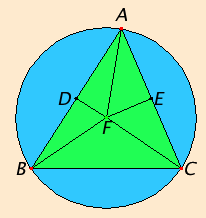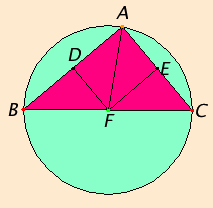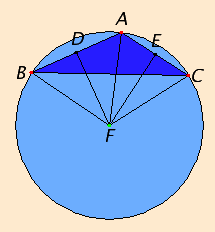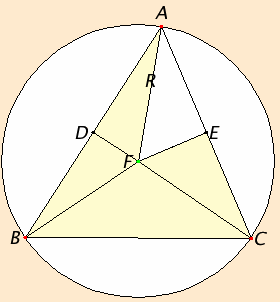# Proposition 5

To circumscribe a circle about a given triangle.

Let ABC be the given triangle.

It is required to circumscribe a circle about the given triangle ABC.Bisect the straight lines AB and AC at the points D and E. Draw DF and EF from the points D and E at right angles to AB and AC. They will then meet within the triangle ABC, or on the straight line BC, or outside BC.

First let them meet within at F. Join FB, FC, and FA.

I.4

Then, since AD equals DB, and DF is common and at right angles, therefore the base AF equals the base FB.

Similarly we can prove that CF also equals AF, so that FB also equals FC, therefore the three straight lines FA and FB and FC equal one another.

IV.Def.6

Therefore the circle described with center F and radius one of the straight lines FA, FB, or FC also passes through the remaining points, and the circle is circumscribed about the triangle ABC.Let it be circumscribed as ABC.

Next, let DF and EF meet on the straight line BC at F, as is the case in the second figure. Join AF.

Then, similarly, we can prove that the point F is the center of the circle circumscribed about the triangle ABC.Next, let DF and EF meet outside the triangle ABC at F, as is the case in the third figure. Join AF, BF, and CF.

I.4

Then again, since AD equals DB, and DF is common and at right angles, therefore the base AF equals the base BF.

IV.Def.6

Similarly we can prove that CF also equals AF, so that BF also equals FC. Therefore the circle described with center F and radius one of the straight lines FA, FB, or FC also passes through the remaining points, and is circumscribed about the triangle ABC.

Therefore a circle has been circumscribed about the given triangle.

Q.E.F.

## Guide

As noted by Simson and others, Euclid does not justify the intersection of the perpendicular bisectors DF and EF. Such a justification is necessary but easy to supply.

The note following the proposition is not actually called a corollary in the Greek text. It is just a remark following the proposition.

#### Circumcircles

This circle drawn about a triangle is called, naturally enough, the circumcircle of the triangle, its center the circumcenter of the triangle, and its radius the circumradius. Much has been discovered about the theory of incircles and circumcircles since Euclid.

 The ratio that appears in the law of sines in trigonometry is the diameter of the circumcircle: 2R = BC / sin A = CA / sin B = AB / sin C where R is the circumradius. This relation is easy to derive from the figure. Angle AFC is twice the angle at B [III.20], but it is also twice angle AFE since the triangles AFE and CFE are congruent. Therefore angle AFE equals the angle at B. Then the sine of B can be found in the right triangle AFE as the ratio of the side AE opposite angle AFE to the hypotenuse AF. Since AE is half of AC, it follows that sin B = AC/(2R) which yields one of the three equations for the law of sines.There is also an equation relating the circumradius R, the inradius r, and the three exradii rA, rB, and rC:

4R = rA + rB + rC – r,

and a number of other interesting results about circumcircles, incircles, and other constructions based on an arbitrary triangle.

This construction is used in propositions IV.10 and XI.23.